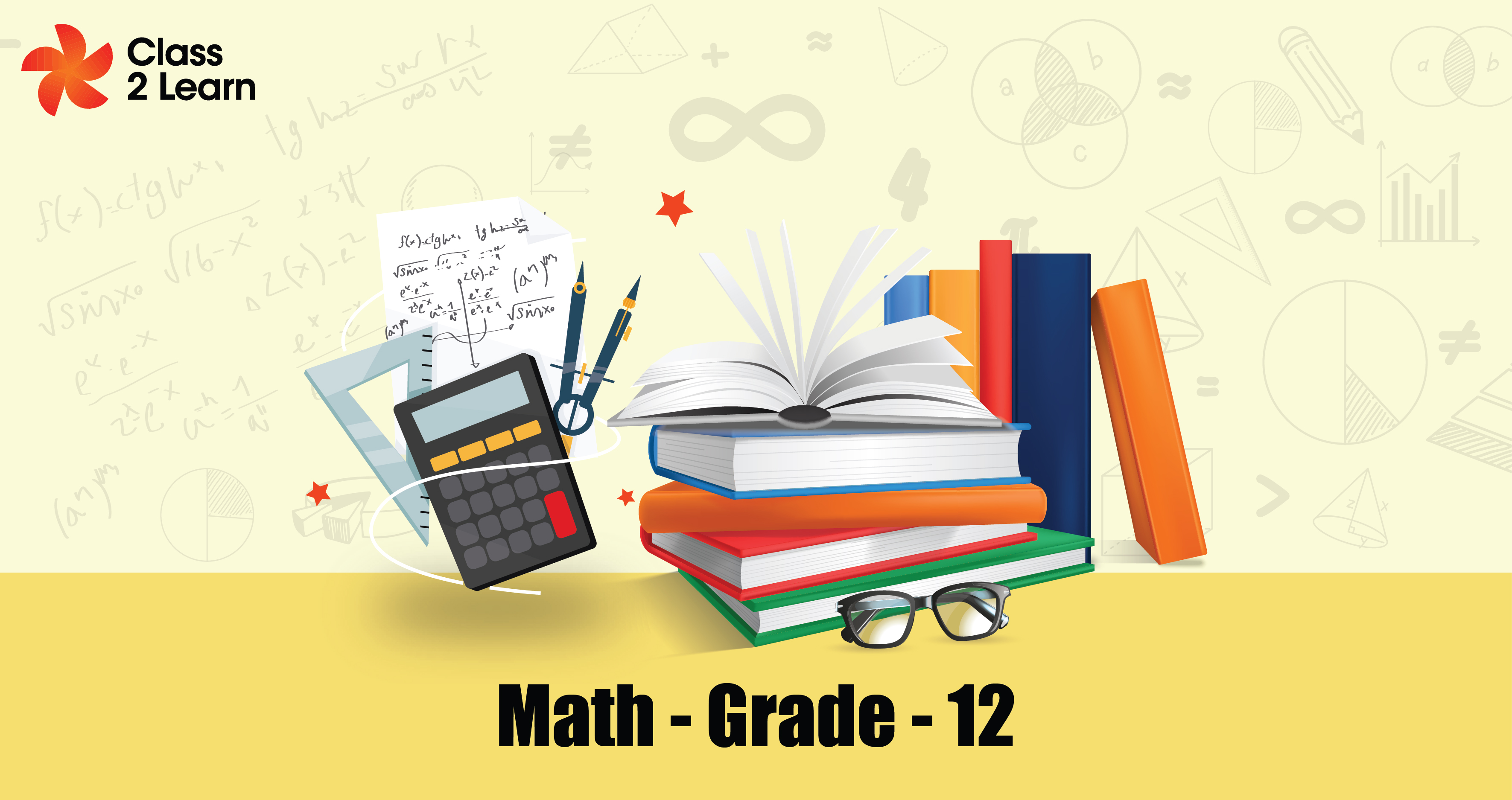# MathematicsMonthly One-time
Price
\$ 10

Per month

#### Overview

Math is an essential subject for all students to learn in order to be prepared for college and their careers. Math helps students develop problem-solving and critical-thinking skills. These skills are valuable in all aspects of life, both personal and

#### Integrals

This chapter guides the student to develop knowledge about Definite integrals and their properties, definite integral formulae, integration using substitution, and integration using trigonometric identities. Students will have expertise in learning through these concepts.

#### APPLICATION OF INTEGRALS AND INVERSE TRIGONOMETRIC FUNCTIONS

This chapter guides the student to develop knowledge about areas of simple curves, area between two curves. The student will gain basic knowledge about inverse trigonometric function and its properties. Students will have expertise in learning through these concepts.

#### DIFFERENTIAL EQUATION

This chapter guides the student to develop knowledge about order and degree, the general form of differential equations. Students will know how to separate the variables using variable separable method and integrate them. They will also learn about Homogeneous differential Equations. Students will have expertise in learning through these concepts.

#### THREE DIMENSIONAL GEOMETRY

This Chapter guides the student to develop knowledge about Three Dimensional geometry involves the mathematics of shapes in 3D space with 3 coordinates in the XYZ plane, which are x-coordinate, y-coordinate, and z-coordinate. Also, the student will learn about the equation of a plane. A plane in three-dimensional space has the equation ax + by + cz + d=0.Linear programming is a method of optimizing operations with some constraints. The main objective of linear programming is to maximize or minimize the numerical value. Students will have expertise in learning through these concepts.

#### Relation and function

This chapter guides the student to develop the skills,, get deep knowledge about equivalence relations, and identify the equivalence class. The student can check whether the given function is bijection or not, and they will learn about composition of functions. Students will know about binary operations and how they are related to basic operations. Students will have expertise in learning these concepts.

#### PROBABILITY

This chapter guides the student to develop knowledge about probability of events, sample space, types of events, probability of random variables and binomial variables, conditional probability of events, permutation, and combination. Students will have expertise in learning through these concepts.

#### Matrices and determinants

This chapter guides the student to develop the skills and get the deep knowledge of knowing basic concepts of matrix and its types, properties, and elementary functions of matrix, meaning of determinants, elementary operations on determinants, and properties and real life applications of determinants. It provides proficiency that will make our students peculiar among all. Students will have expertise in learning through these concepts.

Derivatives

#### Continuity and differentiability

This chapter guides the student to develop the skills and gain deep knowledge in identifying the continuous function, Concept of Rolle’s and mean value theorem, differentiation of parametric function and logarithmic function, and solving problems involving higher order derivatives. Students will have expertise in learning through these concepts.

#### VECTORS

This chapter guides the student to develop knowledge about Vectors-Introduction, vector addition, and vector subtraction). They will learn about cross product, notation and its properties. Also, they will understand the importance of dot products, notation, and properties of vectors. Students will have expertise in learning through these concepts.

#### Application of derivatives

This chapter guides the student to check whether the given function is increasing or decreasing, understand problems related to tangents and normal, find the first and second derivative tests of the function, and find the maxima and minima of the function. Students will gain expertise by learning these concepts.

#### Benefits

##### Included in this plan
• e-books
• Test Series & Analysis
• Assignments & Notes
• Hands on Activity
Free Trial Class

Take advantage of our free trial!